# Constructing a Line Through a Point that is Parallel to a Given Line

Compass and straight edge constructions are of interest to mathematicians, not only in the field of geometry, but also in algebra. For thousands of years, beginning with the Ancient Egyptians, Indians and Greeks, mathematicians were interested in the problem of "duplicating the cube" (constructing a cube with twice the area of a given cube) using a straight edge and compass. It turns out that this is impossible, but no-one managed to prove this until 1837

However, it is possible to construct a line, through a given point, that is parallel to a given line segment using only a pair of compasses and a straight edge.

For this construction, you will need a straight edge (ruler - but you won't be measuring anything), pair of compasses, a pencil and paper. I have drawn the pictures using the robocompass app. It's fun to play with, and you can use it to do all sorts of geometric constructions. There's a little bit of coding to learn, but a list of instructions is provided. Once you've written your little program, you can get out the popcorn, sit back and watch the construction. Be warned that, when you are using it to draw arcs, the robocompass compass point may appear to be a little away from the centre, but the drawing is actually accurate.

## The Construction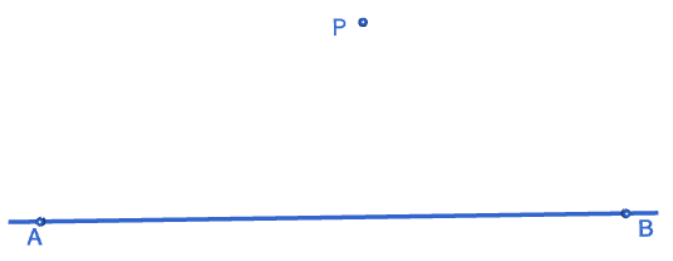Step 1: Start out by drawing the line segment $AB$ and a point $P$ above the line segment through which you want the parallel line segment to pass. Please don't be like Sam! Use a ruler to draw this.Step 2: Using your straight edge (Sam!!!!!), draw a sloping line segment that passes through both $P$ and the line segment $AB$. The angle of the slope is not important, but it's better if it isn't $90^\circ$. Label the point where this line segment cuts the original line segment $J$.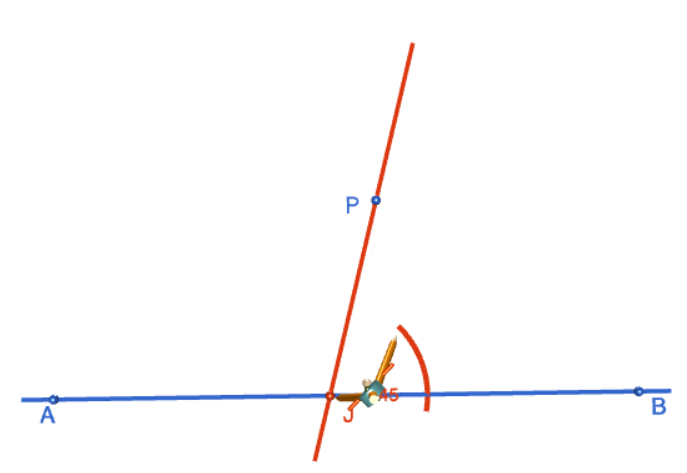Step 3: Open your compasses to a radius that's about half the distance between $P$ and $J$. You're going to draw two arcs of this radius, so please keep this radius fixed. Place the tip of the compasses on $J$, and draw an arc that cuts both line segments $AB$ and $PJ$. The picture shows the tip of the compass a little away from point $J$. This is just a problem with the animation. The arc is actually centred at point $J$, so make sure your compass tip is exactly on point $J$. I have marked the point where the arc cuts the horizontal line by $D$, and the point where it cuts the sloping line by $C$ because we'll need to use them later. The picture on the right shows the completed arcs.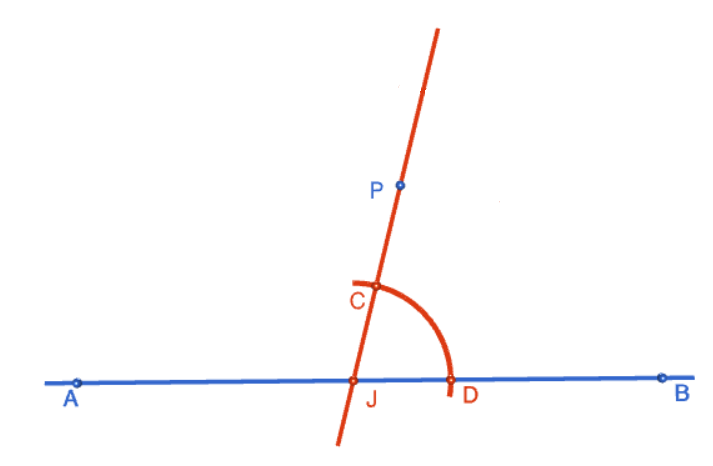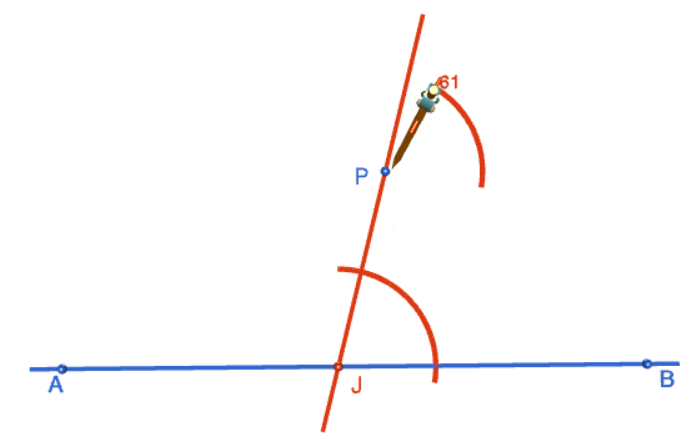Step 4: Move the tip of the compasses up to the point $P$, keeping the same radius. Draw an arc of about the same size as the one you drew down at point $J$, making sure that it also cuts the sloping line. The picture shows the tip of the compasses a little away from point $P$. This is just a problem with the animation. The arc is actually centred at point $P$, so make sure your compass tip is exactly on point $P$. The picture on the right shows the completed arc.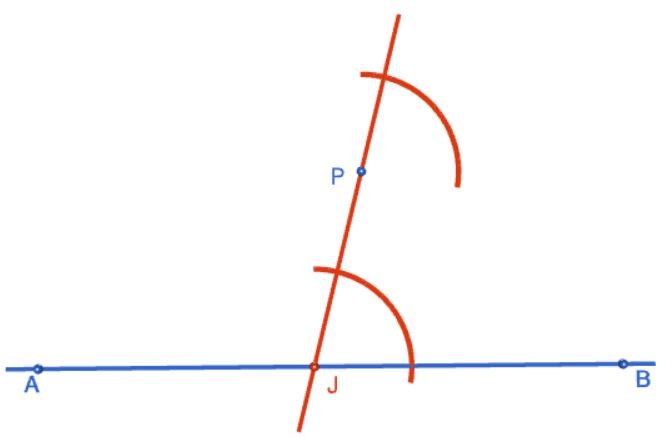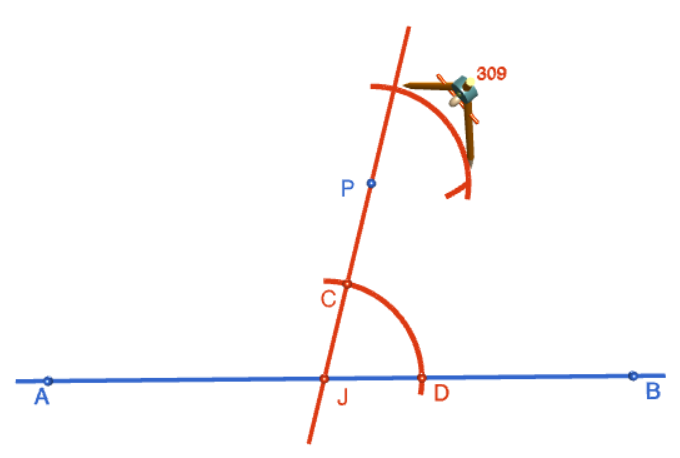Step 5: Open your compasses out to a radius that matches the distance between points $C$ and $D$. Keeping this radius fixed, move the tip of the compasses up to the point where the upper arc crosses the sloping line. Draw an arc, centred at this point of intersection, that crosses the upper arc. The picture on the right shows the completed arc.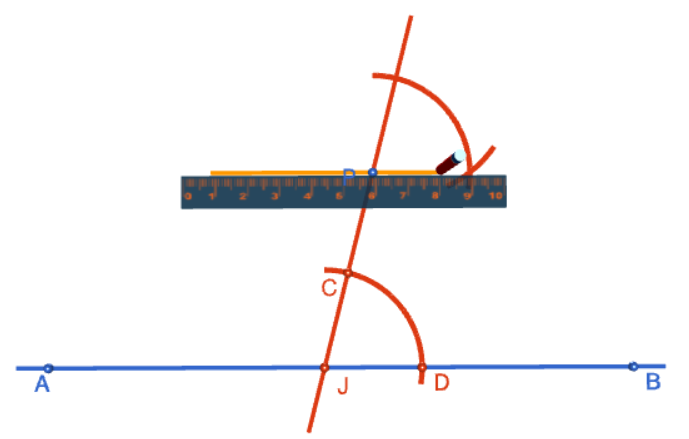Step 6: Use a straight edge to draw a line that passes through the point $P$ and the point where the two upper arcs cross, as shown in the picture on the left. This will be your perpendicular. The picture on the right shows the finished construction. This will be your parallel line. You can check that the two lines are parallel by comparing their corresponding angles using a protractor. Have fun doing the construction!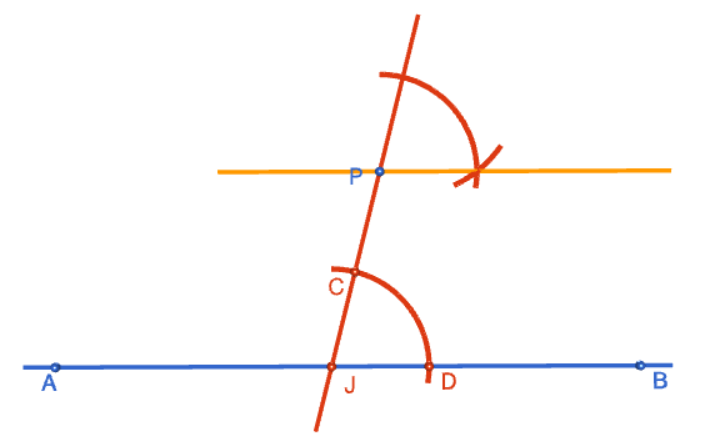### Description

This tutorial will expose you to Rulers and how to use them. We will also have a close look as set square and compass constructions. Your feedback is important to us, if you like any other topic covered under this tutorial, please do let us know.

### Audience

Year 10 or higher

### Learning Objectives

Ruler and compass constructions and more

Author: Subject Coach
You must be logged in as Student to ask a Question.